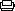## SoylentNews is people

### 128bit Discrete Logarithm Crypto Solved in 2 Hours

posted by LaminatorX on Saturday May 31 2014, @11:48AM[Skip to comment(s)]
from the O-getting-smaller dept.

A class of Discrete Logarithm Problems (DLPs) used in a 128-bit crypto scheme allegedly cracked in two hours (paper). It can also be formulated as breaking 128-bit secure supersingular binary curves or how to solve discrete logarithms fast. Findings to be presented at the IACR Crypto 2014 conference.

This discussion has been archived. No new comments can be posted.
Display Options Threshold/Breakthrough
The Fine Print: The following comments are owned by whoever posted them. We are not responsible for them in any way.
• #### Discrete eh?Discrete eh?(Score: -1, Flamebait) by Anonymous Coward on Saturday May 31 2014, @12:38PM

by Anonymous Coward on Saturday May 31 2014, @12:38PM (#49540)

She said she was discrete but I turn my back for two hours and she spill all my secrets. I knew I couldn't trust that bitch!

#### Re:Discrete eh?(Score: -1, Troll) by Anonymous Coward on Saturday May 31 2014, @01:23PM

by Anonymous Coward on Saturday May 31 2014, @01:23PM (#49550)

That's why you stick it in her pooper then donkey punch her.

• #### Re:Discrete eh?(Score: 2, Informative) by Anonymous Coward on Saturday May 31 2014, @08:32PM

by Anonymous Coward on Saturday May 31 2014, @08:32PM (#49701)

That's what you get for being illiterate.
If only she were discreet...

• #### No WorriesNo Worries(Score: 2) by Zyx Abacab on Sunday June 01 2014, @03:10AM

by Zyx Abacab (3701) on Sunday June 01 2014, @03:10AM (#49798)

From TFA:

Crypto researchers are preparing to scatter the ashes of a class of Discrete Logarithm Problems (DLPs) as the future of security, following a claim by Swiss researchers to have cracked a 128-bit crypto scheme in two hours.

So, no, this doesn't mean that current encryption using 128-bit keys is broken. With sufficient key sizes, AES, Twofish, and Serpent are still not breakable in any reasonable timeframe.

In fact, the research does not show that discrete logarithms are conceptually broken! The only thing that's been demonstrated is that supersingular binary curves, in particular, are not suitable as an encryption scheme.

• #### Re:No WorriesRe:No Worries(Score: 2, Informative) by No.Limit on Sunday June 01 2014, @08:52AM

by No.Limit (1965) on Sunday June 01 2014, @08:52AM (#49855)

AES, twofish & serpent don't even use discrete logarithms. So these ciphers are not affected.

Discrete logarithms can never be 'broken' conceptually. Every crypto that uses discrete logarithms is based on the decisional diffie-hellman assumption [wikipedia.org], which basically states that discrete logarithms are hard to compute. The research shows that this assumption doesn't hold specifically for supersingular curves (which are mathematical groups) with 128 bits. So the research shows that this class of groups isn't suitable for crypto based on discrete logs.

Let's elaborate a little on this:

A & B agree on a common number g (an adversary can see it).
Then A generates a private random x and B generates a private random y.
A -> B k (=g^x)
B -> A m (=g^y)
Then A can compute m^x = (g^y)^x = g^(x*y) and B can compute k^y = (g^x)^y = g^(x*y) so they just shared a private key.

So, about secrecy of this exchange:
An adversary only sees g, k = g^x and m = g^y. If they could find out x or y that is log(g^x) = x (where log is in base g) they find the shared private key.

In this key exchange we've only used exponentiation. Let's say multiplication can be done in constant time, then we can compute exponentiation g^n in asymptotic time complexity O(log(n)) (now with any base) with the fast powering method [wikipedia.org].

Well for real numbers we can use that u diffie-hellman key exchange), after which the secret shared key can be used as key to any cipher such as AES, twofish, serpent etc.

• #### Re:No Worries(Score: 2, Informative) by No.Limit on Sunday June 01 2014, @08:59AM

by No.Limit (1965) on Sunday June 01 2014, @08:59AM (#49856)

Ignore the last paragraph, because of the smaller equal a big chunk of text got missing. It should continue like this:

Well for real numbers we can use that

u <= v is equivalent to g^u <= g^v

and use a binary search (first expanding and then narrowing) to compute it in O(log(n)).

However in cyclic groups this does not hold (in cyclic groups the notion of u smaller v doesn't make much sense).
So we can't use the above trick. Let's say we're unlucky and are only left with a brute-force trial and error which would take O(n) time.

Now we have O(log(n)) for exponentiation and O(n) for logarithm. We set n' = log(n) and get O(n') for exponentiation and O(e^n') for logarithm.
And that's the relationship we're using to make sure that no adversary has enough computational power to successfully compute the logarithm.

With this we just need groups where the logarithm can't be computed efficiently. Well supersingular curves probably aren't suitable.

Now if P = NP we can show that no such group exists. In fact with P = NP we can break any practical scheme and would be left with OTP as the most efficient crypto. Luckily P = NP is a very hard unsolved mathematical problem and there are still many classes of groups where the decisional diffie-hellman assumption is believed to hold true.

Btw the name discrete logarithm comes because these groups aren't continuous like the real numbers. They can be represented by integers.

Discrete logarithms are often used for key exchange (for example in the diffie-hellman key exchange [wikipedia.org]), after which the secret shared key can be used as key to any cipher such as AES, twofish, serpent etc.